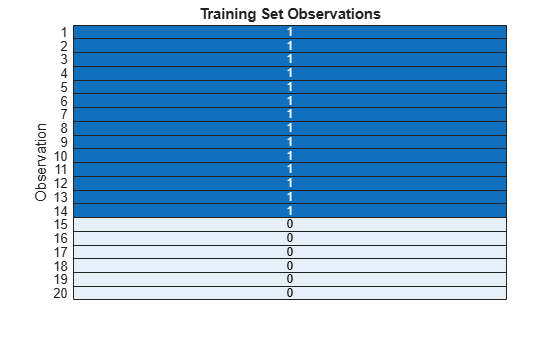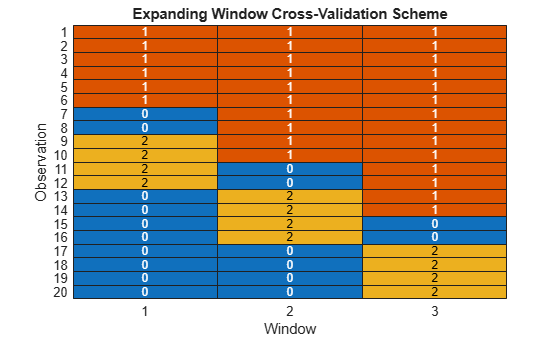# training

Training indices for time series cross-validation

Since R2022b

## Syntax

``idx = training(c)``
``idx = training(c,i)``

## Description

example

````idx = training(c)` returns the training indices `idx` for a `tspartition` object `c` of type `'holdout'`. That is, the logical vector `idx` specifies the observations in the training set.```

example

````idx = training(c,i)` returns the training indices for window `i` of a `tspartition` object `c` of type `'expanding-window'` or `'sliding-window'`. That is, the logical vector `idx` specifies the observations in training set `i`.If `c.Type` is `'expanding-window'`, then the training set size expands with each window while the test set size remains fixed.If `c.Type` is `'sliding-window'`, then both the training set size and the test set size are fixed.```

## Examples

collapse all

Identify the observations in the training set of a `tspartition` object for holdout validation.

Use 30% of 20 time-dependent observations to create a test set. The corresponding training set contains the remaining observations.

`c = tspartition(20,"Holdout",0.30);`

Find the training set indices. A value of 1 (`true`) indicates that the corresponding observation is in the training set. A value of 0 (`false`) indicates that the corresponding observation is in the test set.

`trainingIndices = training(c);`

Visualize the observations in the training set by using a heat map.

```h = heatmap(double(trainingIndices),ColorbarVisible="off"); h.XDisplayLabels = ""; ylabel("Observation") title("Training Set Observations")```The observations in dark blue (with a value of 1) are in the training set, and the observations in light blue (with a value of 0) are in the test set. When you use holdout validation for time series data, the latest observations (in this case, observations 15 through 20) are in the test set.

Identify the observations in the training sets and test sets of a `tspartition` object for expanding window cross-validation.

Use 20 time-dependent observations to create three training sets and three test sets. Specify a gap of two observations between each training set and its corresponding test set.

```c = tspartition(20,"ExpandingWindow",3, ... GapSize=2);```

Find the training set indices for the three windows. A value of 1 (`true`) indicates that the corresponding observation is in the training set for that window.

```trainWindow1 = training(c,1); trainWindow2 = training(c,2); trainWindow3 = training(c,3);```

Find the test set indices for the three windows. A value of 1 (`true`) indicates that the corresponding observation is in the test set for that window.

```testWindow1 = test(c,1); testWindow2 = test(c,2); testWindow3 = test(c,3);```

Combine the training and test set indices into one matrix where a value of 1 indicates a training observation and a value of 2 indicates a test observation.

```data = [trainWindow1 + 2*testWindow1, ... trainWindow2 + 2*testWindow2, ... trainWindow3 + 2*testWindow3];```

Visualize the different sets by using a heat map.

```colormap = lines(3); heatmap(double(data),ColorbarVisible="off", ... Colormap=colormap); xlabel("Window") ylabel("Observation") title("Expanding Window Cross-Validation Scheme")```For each window, the observations in red (with a value of 1) are in the training set, the observations in yellow (with a value of 2) are in the test set, and the observations in blue (with a value of 0) are ignored. For example, observation 11 is a test observation in window one, a gap observation in window two, and a training observation in window three.

## Input Arguments

collapse all

Time series validation partition, specified as a `tspartition` object. The validation partition type (`Type`) is `'expanding-window'`, `'holdout'`, or `'sliding-window'`.

Training set or window index, specified as a positive integer scalar. When you specify `i`, the `training` function finds the observations in training set `i`.

Data Types: `single` | `double`

## Output Arguments

collapse all

Indices for training set observations, returned as a logical vector. A value of 1 (`true`) indicates that the corresponding observation is in the training set. A value of 0 (`false`) indicates that the corresponding observation is in a different set, such as the test set.

## Version History

Introduced in R2022b